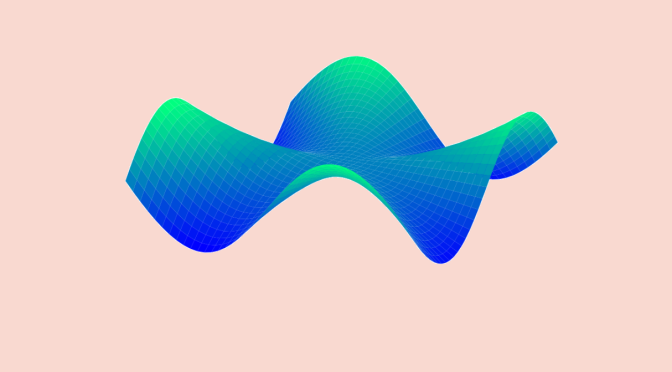# Differentiability of multivariable real functions (part2)

Following the article on differentiability of multivariable real functions (part 1), we look here at second derivatives. We consider a function $$f : \mathbb R^n \to \mathbb R$$ with $$n \ge 2$$.

Schwarz’s theorem states that if $$f : \mathbb R^n \to \mathbb R$$ has continuous second partial derivatives at any given point in $$\mathbb R^n$$, then for $$(a_1, \dots, a_n) \in \mathbb R^n$$ and $$i,j \in \{1, \dots, n\}$$:
$\frac{\partial^2 f}{\partial x_i \partial x_j}(a_1, \dots, a_n)=\frac{\partial^2 f}{\partial x_j \partial x_i}(a_1, \dots, a_n)$

### A function for which $$\frac{\partial^2 f}{\partial x \partial y}(0,0) \neq \frac{\partial^2 f}{\partial y \partial x}(0,0)$$

We consider:
$\begin{array}{l|rcl} f : & \mathbb R^2 & \longrightarrow & \mathbb R \\ & (0,0) & \longmapsto & 0\\ & (x,y) & \longmapsto & \frac{xy(x^2-y^2)}{x^2+y^2} \text{ for } (x,y) \neq (0,0) \end{array}$

$$f$$ is defined and indefinitely differentiable at all points different from the origin. For $$(x,y) \neq (0,0)$$:
\begin{align*} \left\vert \frac{f(x,y)}{\sqrt{x^2+y^2}} \right\vert & = \left\vert \frac{xy(x^2-y^2)}{\sqrt{x^2+y^2}(x^2+y^2)} \right\vert\\
& \le \left\vert \frac{xy}{\sqrt{x^2+y^2}} \right\vert\\
& \le \frac{(\vert x \vert + \vert y \vert)^2}{2 \sqrt{x^2+y^2}}\\
& \le \sqrt{x^2+y^2}\end{align*} as we have for all $$(x,y) \in \mathbb R^2$$: $$\vert xy \vert \le \frac{(\vert x \vert +\vert y \vert)^2}{2}$$ and $$(\vert x \vert +\vert y \vert)^2 \le 2 (x^2+y^2)$$. This proves that $$f$$ is continuous and differentiable at the origin, with $$f(0,0)=0$$ and a Fréchet derivative equal to zero at the origin. So $\frac{\partial f}{\partial x}(0,0)=\frac{\partial f}{\partial y}(0,0)=0$ We now look at the mixed partial derivatives.

For $$(x,y) \neq (0,0)$$:$\begin{cases} \frac{\partial f}{\partial x}(x,y) = \frac{(x^2+y^2)(3x^2y-y^3)+2x^2y(y^2-x^2)}{(x^2+y^2)^2}\\ \frac{\partial f}{\partial y}(x,y) = \frac{(x^2+y^2)(x^3-3x y^2)+2xy^2(y^2-x^2)}{(x^2+y^2)^2} \end{cases}$ In particular:
\begin{align*}
\frac{\partial f}{\partial x}(0,y) &= -y \text{ for } y \neq 0\\
\frac{\partial f}{\partial x}(x,0) &= x \text{ for } x \neq 0
\end{align*} which implies $\frac{\partial^2 f}{\partial x \partial y}(0,0) =1 \neq -1 = \frac{\partial^2 f}{\partial y \partial x}(0,0)$
The mixed second derivatives of $$f$$ are not symmetric.